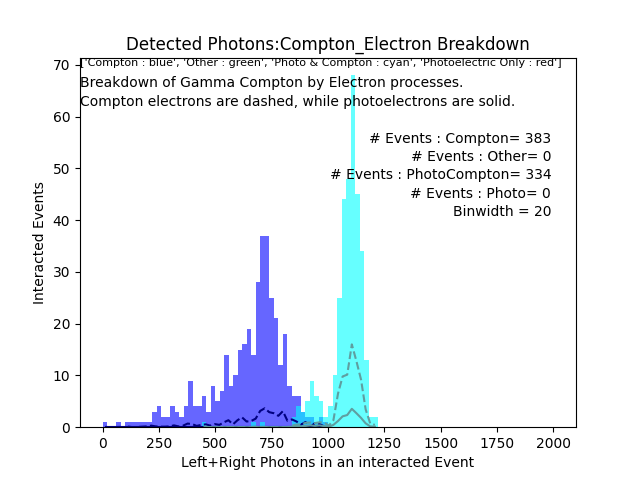# Gamma photoelectric/compton processes & electron creator processes do not produce similar histograms

I am simulating a PET scanner, and need to validate my energy histograms by separating them by process (Photoelectric vs Compton scatter).

When I do this via gamma step processes, I get a different result than via electron creator processes. As far as I understand the physics, they should be nearly symmetric!

Setup: 511keV gamma fired into detector composed of EJ208 (PVT scintillator) and ESR film (reflective foil).

Let :

• A gamma photoelectric event be defined as one in which the gamma only interacts via photoelectric interactions. (Red in plot)
• A gamma compton event be defined as one in which the gamma only interacts via compton interactions. (Blue in plot)
• A gamma photocompton event is defined as an event in which the gamma interacts with the detector via both compton and photoelectric interactions. (Cyan in plot)
``````ps = postPoint->GetProcessDefinedStep();
if((ps) && (step->GetStepLength()>0))
{
G4String psNm = ps->GetProcessName();

if(psNm.compare("phot")==0)
{..... }
else if(psNm.compare("compt")==0)
{..... }
....
}
``````

Let :

• An electron photoelectric event be defined as one in which all electrons are produced via photoelectric interactions. (Red in plot)
• An electron compton event be defined as one in which all electrons are produced via compton interactions. (Blue in plot)
• An electron photocompton event is defined as an event in which electrons are produced via both compton and photoelectric interactions. (Cyan in plot)
`````` 	const std::vector< const G4Track* >* secondaries = step->GetSecondaryInCurrentStep();
const int size = secondaries->size();
const std::string title[] = { "e+","e-","opticalphoton","gamma","proton","alpha","Li6","Be7","C11","C12","N15","O15","O16"};
const int titleSize = sizeof(title)/sizeof(title);

G4double Esec = (edep);
const G4VProcess* ps;

for(int n = 0; n < size; n++)
{
G4Track* ptrG4Track = (G4Track*)(*secondaries)[n];
const G4DynamicParticle* pp = (const G4DynamicParticle*) ptrG4Track->GetDynamicParticle();
const G4ParticleDefinition* pd = (const G4ParticleDefinition*) pp->GetParticleDefinition();
const std::string pdVar = pd->GetParticleName();
if(pdVar.compare("e-")==0)
{
fEventAction->electronProcessName.push_back(ptrG4Track->GetCreatorProcess()->GetProcessName()); // electron process name;
fEventAction->electronProcess.push_back(ptrG4Track->GetTrackID()); // eventID, trackID
}
.....
}
``````

Gamma Processes Breakdown:

Electron Creator Process Breakdown:

(Note that the other (green) category is currently not considered.)
(Also please ignore the count discrepancy, this is not due to low statistics, since the total histograms are nearly identical.)

Clearly, the electron graph makes the most physical sense.
The gamma is also recorded as having multiple photoelectric interactions - the only way this is possible is if the gamma was re-emitted by the photoelectron! Am I counting the gamma processes correctly?

If I were to breakdown the “gamma compton” events by electron creator process (events in which the gamma only interacted via compton interactions):Somehow I get nonzero energetic photoelectrons (the cyan peak) in an event where the gamma has only interacted via compton scattering!

As far as I understand, this must be a code error, since there is no way for a compton electron to emit a photon that then produces a photoelectric effect with high enough energy to create the cyan peak.

Any help would be greatly appreciated.

Thank you so much,
Akhil

Would really appreciate any suggestions. Is there at least an example that someone could point me to?
Thank you.

P.S. (If this post is in an incorrect category, please let me know.)

Hello,

are fluorescence and Auger electron emission enabled in this tests?

VI